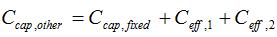# HOMER Pro 3.15Type: Output Variable Units: \$ Symbol: Ccap,other

HOMER uses the Other capital cost variable to account for the system fixed capital cost and, if any primary load efficiency measures are in effect for the current system configuration, the capital cost associated with those efficiency measures.

The Other capital cost variable appears in the Cash Flow table under the Cash Flow tab in the Simulation Results window.

HOMER uses the following equation to calculate the Other capital cost:where: Ccap,fixed Ceff,1 = cost of efficiency measures (if any) for primary load 1 [\$] Ceff,2 = cost of efficiency measures (if any) for primary load 2 [\$]